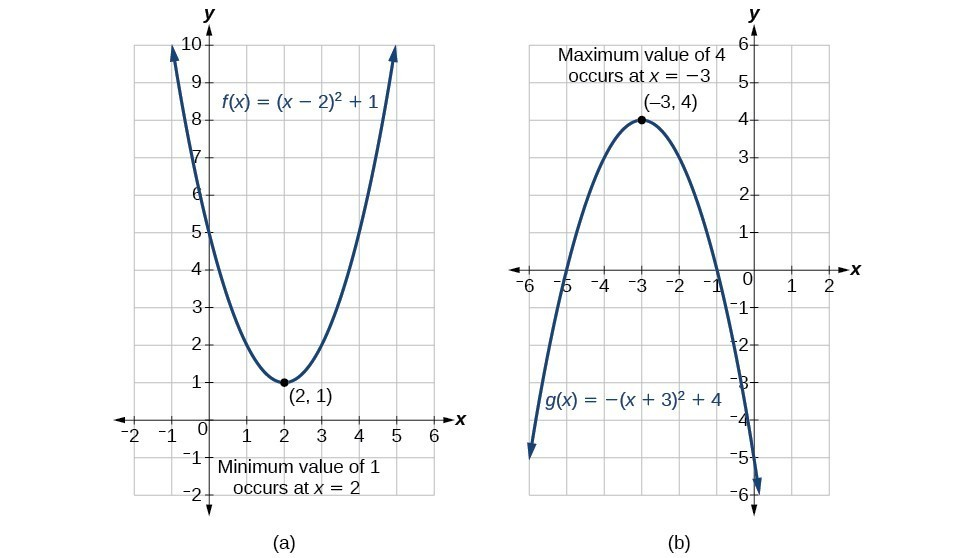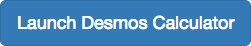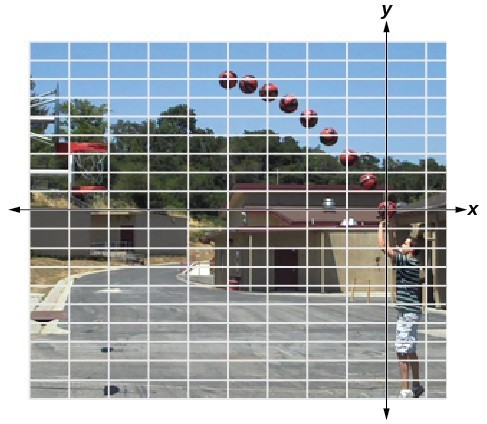## 10.3 – Applications of Quadratic Functions

### Learning Objectives

• (10.3.1) – Solve application problems involving quadratic functions
• Objects in free fall
• Determining the width of a border
• Finding the maximum and minimum values of a quadratic function

# (10.3.1) – Solve application problems involving quadratic functions

Quadratic equations are widely used in science, business, and engineering. Quadratic equations are commonly used in situations where two things are multiplied together and they both depend on the same variable. For example, when working with area, if both dimensions are written in terms of the same variable, you use a quadratic equation. Because the quantity of a product sold often depends on the price, you sometimes use a quadratic equation to represent revenue as a product of the price and the quantity sold. Quadratic equations are also used when gravity is involved, such as the path of a ball or the shape of cables in a suspension bridge.

### Objects in free fall

A very common and easy-to-understand application is the height of a ball thrown at the ground off a building. Because gravity will make the ball speed up as it falls, a quadratic equation can be used to estimate its height any time before it hits the ground. Note: The equation isn’t completely accurate, because friction from the air will slow the ball down a little. For our purposes, this is close enough.

### Example

A ball is thrown off a building from 200 feet above the ground. Its starting velocity (also called initial velocity) is $−10$ feet per second. (The negative value means it’s heading toward the ground.)

The equation $h=-16t^{2}-10t+200$ can be used to model the height of the ball after $t$ seconds. About how long does it take for the ball to hit the ground?

In the next video we show another example of how the quadratic equation can be used to find the time it takes for an object in free fall to hit the ground.

Here are some more similar objects in free fall examples.

### Example: Applying the Vertex and $x$-Intercepts of a Parabola

A ball is thrown upward from the top of a 40 foot high building at a speed of 80 feet per second. The ball’s height above ground can be modeled by the equation $H\left(t\right)=-16{t}^{2}+80t+40$.

a. When does the ball reach the maximum height?

b. What is the maximum height of the ball?

c. When does the ball hit the ground?

### EXAMPLE

A rock is thrown upward from the top of a 112-foot high cliff overlooking the ocean at a speed of 96 feet per second. The rock’s height above ocean can be modeled by the equation $H\left(t\right)=-16{t}^{2}+96t+112$.

a. When does the rock reach the maximum height?

b. What is the maximum height of the rock?

c. When does the rock hit the ocean?

### Applications of quadratic functions: determining the width of a border

The area problem below does not look like it includes a Quadratic Formula of any type, and the problem seems to be something you have solved many times before by simply multiplying. But in order to solve it, you will need to use a quadratic equation.

### Example

Bob made a quilt that is 4 ft $\times$ 5 ft. He has 10 sq. ft. of fabric he can use to add a border around the quilt. How wide should he make the border to use all the fabric? (The border must be the same width on all four sides.)

Here is a video which gives another example of using the quadratic formula for a geometry problem involving the border around a quilt.

### Finding the maximum and minimum values of a quadratic function

There are many real-world scenarios that involve finding the maximum or minimum value of a quadratic function, such as applications involving area and revenue.### ExAMPLE

Find two numbers $x$ and $y$ whose difference is 100 and whose product is a minimum.

### Example: Finding the Maximum Value of a Quadratic Function

A backyard farmer wants to enclose a rectangular space for a new garden within her fenced backyard. She has purchased 80 feet of wire fencing to enclose three sides, and she will use a section of the backyard fence as the fourth side.

1. Find a formula for the area enclosed by the fence if the sides of fencing perpendicular to the existing fence have length $L$.
2. What dimensions should she make her garden to maximize the enclosed area?

### How To: Given an application involving revenue, use a quadratic equation to find the maximum.

1. Write a quadratic equation for revenue.
2. Find the vertex of the quadratic equation.
3. Determine the $y$-value of the vertex.

### Example: Finding Maximum Revenue

The unit price of an item affects its supply and demand. That is, if the unit price goes up, the demand for the item will usually decrease. For example, a local newspaper currently has 84,000 subscribers at a quarterly charge of $30. Market research has suggested that if the owners raise the price to$32, they would lose 5,000 subscribers. Assuming that subscriptions are linearly related to the price, what price should the newspaper charge for a quarterly subscription to maximize their revenue?

### Try It

A coordinate grid has been superimposed over the quadratic path of a basketball in the picture below. Find an equation for the path of the ball. Does the shooter make the basket?(credit: modification of work by Dan Meyer)